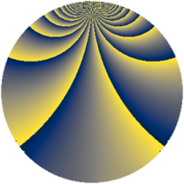# Properties

 Label 1470.2.beLevel $1470$ Weight $2$ Character orbit 1470.be Rep. character $\chi_{1470}(41,\cdot)$ Character field $\Q(\zeta_{14})$ Dimension $432$ Sturm bound $672$

# Related objects

## Defining parameters

 Level: $$N$$ $$=$$ $$1470 = 2 \cdot 3 \cdot 5 \cdot 7^{2}$$ Weight: $$k$$ $$=$$ $$2$$ Character orbit: $$[\chi]$$ $$=$$ 1470.be (of order $$14$$ and degree $$6$$) Character conductor: $$\operatorname{cond}(\chi)$$ $$=$$ $$147$$ Character field: $$\Q(\zeta_{14})$$ Sturm bound: $$672$$

## Dimensions

The following table gives the dimensions of various subspaces of $$M_{2}(1470, [\chi])$$.

Total New Old
Modular forms 2064 432 1632
Cusp forms 1968 432 1536
Eisenstein series 96 0 96

## Trace form

 $$432q + 72q^{4} + 14q^{6} - 12q^{7} + 10q^{9} + O(q^{10})$$ $$432q + 72q^{4} + 14q^{6} - 12q^{7} + 10q^{9} - 72q^{16} + 16q^{18} + 16q^{21} - 72q^{25} - 84q^{27} + 12q^{28} + 4q^{30} - 10q^{36} + 116q^{37} + 24q^{39} - 80q^{42} - 40q^{43} + 60q^{46} + 56q^{49} + 24q^{51} + 84q^{52} - 40q^{57} + 40q^{58} + 252q^{61} - 20q^{63} + 72q^{64} + 88q^{67} - 56q^{69} + 12q^{70} - 16q^{72} + 32q^{78} + 24q^{79} + 30q^{81} - 16q^{84} + 112q^{87} - 80q^{91} - 8q^{93} - 112q^{94} + 256q^{99} + O(q^{100})$$

## Decomposition of $$S_{2}^{\mathrm{new}}(1470, [\chi])$$ into newform subspaces

The newforms in this space have not yet been added to the LMFDB.

## Decomposition of $$S_{2}^{\mathrm{old}}(1470, [\chi])$$ into lower level spaces

$$S_{2}^{\mathrm{old}}(1470, [\chi]) \cong$$ $$S_{2}^{\mathrm{new}}(147, [\chi])$$$$^{\oplus 4}$$$$\oplus$$$$S_{2}^{\mathrm{new}}(294, [\chi])$$$$^{\oplus 2}$$$$\oplus$$$$S_{2}^{\mathrm{new}}(735, [\chi])$$$$^{\oplus 2}$$